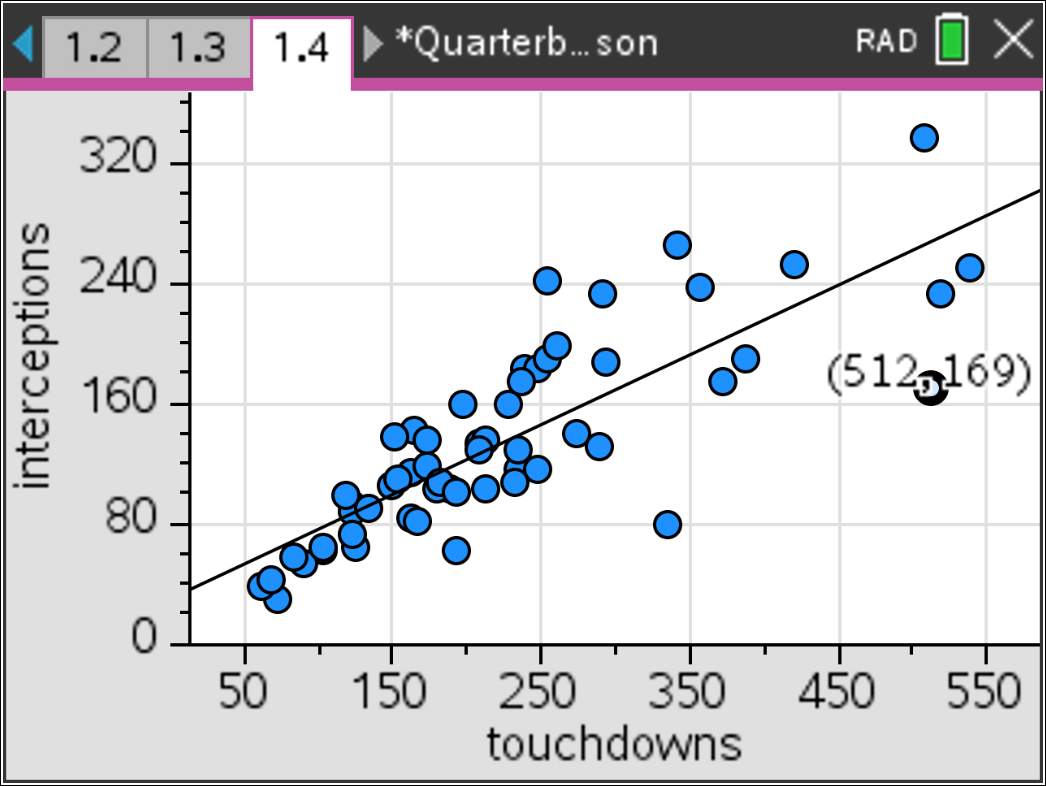# Activities

••• ##### Subject Area

• Math: Mathematical Modeling: Ratings

6-8
9-12

50 Minutes

• ##### Device
•TI-Nspire™ CX/CX II
•TI-Nspire™ CX CAS/CX II CAS
• ##### Software

TI-Nspire™ CX
TI-Nspire™ CX CAS

5.0

## Modeling QB Passing Rates#### Activity Overview

This activity uses the measures commonly collected for football quarterbacks and provides an opportunity for students to create their own quarterback rating models.

#### Objectives

• Students will model a contextual situation mathematically and use the model to answer a question.
• Students will represent data on two quantitative variables on a scatter plot, and describe how the variables are related.
• Students will find and interpret linear equations to model relationships between two quantitative variables.
• Students will use proportional relationships to solve real-world and mathematical problems.